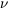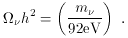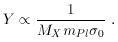### 5. DARK MATTER

5.1 WIMPY Thermal Relics

In this school, the matter of neutrino masses has been reviewed in great detail. A neutrino of mass mcontributes toh2 an amount(12)

If the mass of the neutrino is significantly less than 0.1 eV, then its contribution to0 is dynamically unimportant.

More promising than neutrino hot dark matter is cold dark matter. The most promising candidate for cold dark matter is the lightest supersymmetric particle, presumably a neutralino. Neutralino dark matter has been well studied and reviewed .

The next most popular dark-matter candidate is the axion. Although the axion is very light, since its origin is from a condensate, it is very cold. Axion dark matter has also been well studied and well reviewed .

There are presently several experiments searching for cosmic neutralinos and cosmic axions. Both types of searches seem sensitive enough to discover the relic dark matter, although it will take quite some time (and probably another generation of experiments) to completely cover the parameter space.

Neutralinos are an example of a thermal relic. A thermal relic is assumed to be in local thermodynamic equilibrium (LTE) at early times. The equilibrium abundance of a particle, say relative to the entropy density, depends upon the ratio of the mass of the particle to the temperature. If we define the variables YnX / s and x = MX / T, where nX is the number density of WIMP (weakly interacting massive particle) X with mass MX and s ~ T3 is the entropy density, Yexp(-x) for x >> 1, while Y ~ constant for x << 1.

A particle will track its equilibrium abundance so long as reactions which keep the particle in chemical equilibrium can proceed rapidly enough. Here, rapidly enough means on a timescale more rapid than the expansion rate of the universe H. When the reactions becomes slower than the expansion rate, then the particle can no longer track its equilibrium value and thereafter Y is constant. When this occurs, the particle is said to be ``frozen out.'' A schematic illustration of this is given in Fig. 8.Figure 8. A thermal relic starts in LTE at T >> MX. When the rates keeping the relic in chemical equilibrium become smaller than the expansion rate, the density of the relic relative to the entropy density becomes constant. This is known as freeze out.

The more strongly interacting the particle, the longer it stays in LTE, and the smaller its freeze-out abundance. Thus, the more weakly interacting the particle, the larger its present abundance. The freeze-out value of Y is related to the mass of the particle and its annihilation cross section (here characterized by0) by(13)

Since the contribution tois proportional to MX nX, which in turn is proportional to MXY, the present contribution tofrom a thermal relic is (to first approximation) independent of the mass, and only depends on the mass indirectly through the dependence of the annihilation cross section on mass. The largest that the annihilation cross section can be is roughly MX-2. This implies that large-mass WIMPS would have such a small annihilation cross section that their present abundance would be too large. Thus, one expects a maximum mass for a thermal WIMP, which turns out to be a few hundred TeV.

The mass of WIMPS usually considered for dark matter run from a microvolt for axions to several dozen GeV for neutralinos. With the exception of massive magnetic monopoles, the possibility of dark matter particles of GUT-scale mass is not usually considered, because thermal relics of this mass would be expected to be over abundant by several orders of magnitude.

Recently, the idea that dark matter may be supermassive has received a lot of attention. Since wimpy little dark matter particles with mass less than a TeV are called WIMPS, dark matter particles of really hefty mass of 1012 to 1016 GeV seem to be more than WIMPS, so they are referred to as WIMPZILLAS.